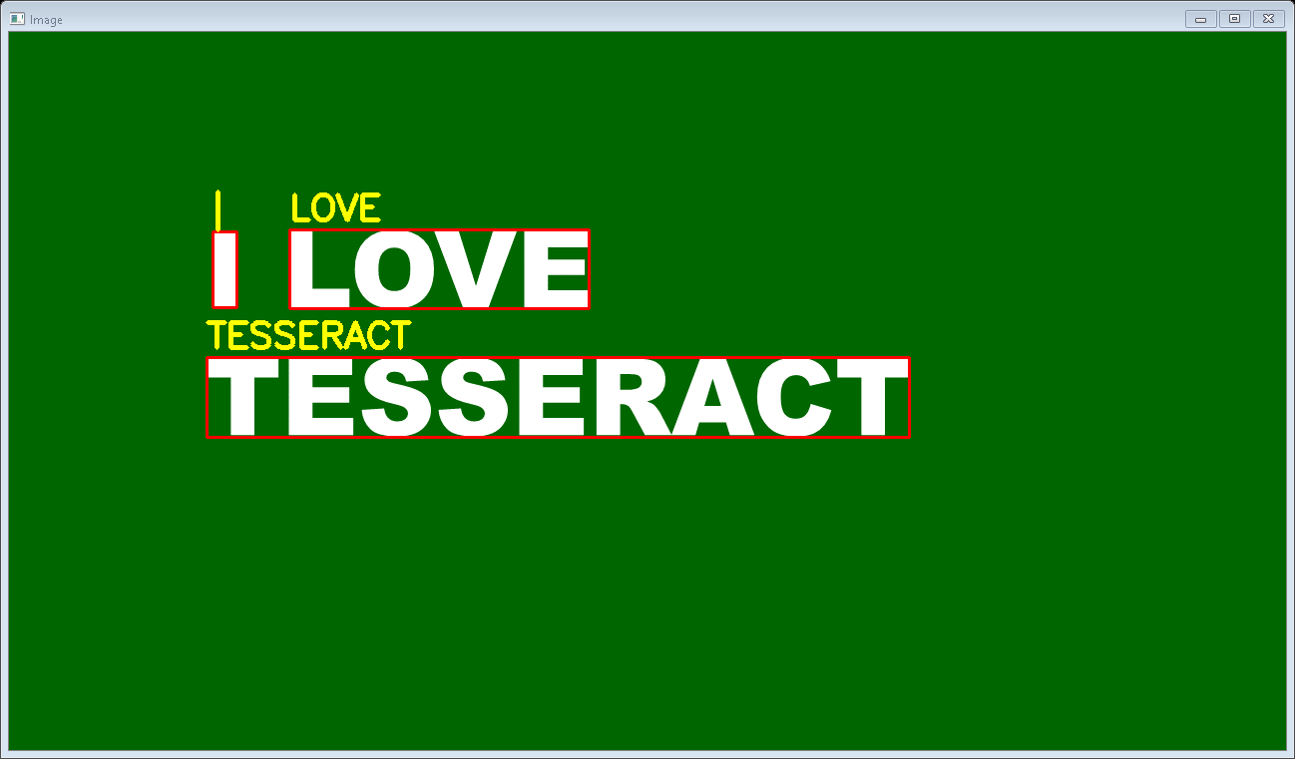# Text Localization, Detection and Recognition using Pytesseract

Pytesseract or Python-tesseract is an Optical Character Recognition (OCR) tool for Python. It will read and recognize the text in images, license plates etc. Python-tesseract is actually a wrapper class or a package for Google’s Tesseract-OCR Engine. It is also useful and regarded as a stand-alone invocation script to tesseract, as it can easily read all image types supported by the Pillow and Leptonica imaging libraries, which mainly includes –

• jpg
• png
• gif
• bmp
• tiff etc

Also additionally, if it is used as a script, Python-tesseract will also print the recognized text instead of writing it to a file. Python-tesseract can be installed using pip as shown below –

`pip install pytesseract`

If you are using Anaconda Cloud, Python-tesseract can be installed as shown below:-

`conda install -c conda-forge/label/cf202003 pytesseract`

or

`conda install -c conda-forge pytesseract`

Note: tesseract should be installed in the system before running the below script.
Below is the implementation.

## Python3

 `from` `pytesseract ``import``*` `import` `argparse ` `import` `cv2 ` ` `  ` `  `# We construct the argument parser ` `# and parse the arguments ` `ap ``=` `argparse.ArgumentParser() ` ` `  `ap.add_argument(``"-i"``, ``"--image"``, ` `                ``required``=``True``, ` `                ``help``=``"path to input image to be OCR'd"``) ` `ap.add_argument(``"-c"``, ``"--min-conf"``, ` `                ``type``=``int``, default``=``0``, ` `                ``help``=``"minimum confidence value to filter weak text detection"``) ` `args ``=` `vars``(ap.parse_args()) ` ` `  `# We load the input image and then convert ` `# it to RGB from BGR. We then use Tesseract ` `# to localize each area of text in the input ` `# image ` `images ``=` `cv2.imread(args[``"image"``]) ` `rgb ``=` `cv2.cvtColor(images, cv2.COLOR_BGR2RGB) ` `results ``=` `pytesseract.image_to_data(rgb, output_type``=``Output.``DICT``) ` ` `  `# Then loop over each of the individual text ` `# localizations ` `for` `i ``in` `range``(``0``, ``len``(results[``"text"``])): ` `     `  `    ``# We can then extract the bounding box coordinates ` `    ``# of the text region from  the current result ` `    ``x ``=` `results[``"left"``][i] ` `    ``y ``=` `results[``"top"``][i] ` `    ``w ``=` `results[``"width"``][i] ` `    ``h ``=` `results[``"height"``][i] ` `     `  `    ``# We will also extract the OCR text itself along ` `    ``# with the confidence of the text localization ` `    ``text ``=` `results[``"text"``][i] ` `    ``conf ``=` `int``(results[``"conf"``][i]) ` `     `  `    ``# filter out weak confidence text localizations ` `    ``if` `conf > args[``"min_conf"``]: ` `         `  `        ``# We will display the confidence and text to ` `        ``# our terminal ` `        ``print``(``"Confidence: {}"``.``format``(conf)) ` `        ``print``(``"Text: {}"``.``format``(text)) ` `        ``print``("") ` `         `  `        ``# We then strip out non-ASCII text so we can ` `        ``# draw the text on the image We will be using ` `        ``# OpenCV, then draw a bounding box around the ` `        ``# text along with the text itself ` `        ``text ``=` `"".join(text).strip() ` `        ``cv2.rectangle(images, ` `                      ``(x, y), ` `                      ``(x ``+` `w, y ``+` `h), ` `                      ``(``0``, ``0``, ``255``), ``2``) ` `        ``cv2.putText(images, ` `                    ``text, ` `                    ``(x, y ``-` `10``),  ` `                    ``cv2.FONT_HERSHEY_SIMPLEX, ` `                    ``1.2``, (``0``, ``255``, ``255``), ``3``) ` `         `  `# After all, we will show the output image ` `cv2.imshow(``"Image"``, images) ` `cv2.waitKey(``0``) `

Output:
Execute the command below to view the Output

`python ocr.py --image ocr.png `In addition to Output, we will see the Confidence Level and the Text In Command Prompt as shown below –

```Confidence: 93
Text: I

Confidence: 93
Text: LOVE

Confidence: 91
Text: TESSERACT```

Whether you're preparing for your first job interview or aiming to upskill in this ever-evolving tech landscape, GeeksforGeeks Courses are your key to success. We provide top-quality content at affordable prices, all geared towards accelerating your growth in a time-bound manner. Join the millions we've already empowered, and we're here to do the same for you. Don't miss out - check it out now!

Previous
Next